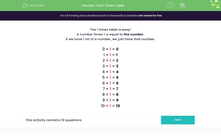# The 1 Times Table

In this worksheet, students answer questions on the 1 times table!Key stage:  KS 1

Curriculum topic:   Number: Multiplication and Division

Curriculum subtopic:   Solve Multiplication/Division Problems

Popular topics:   Times Table worksheets

Difficulty level:#### Worksheet Overview

The 1 times table is easy!

A number times 1 is equal to the number.

If we have 1 lot of a number, we just have that number.

0 × 1 = 0

1 × 1 = 1

2 × 1 = 2

3 × 1 = 3

4 × 1 = 4

5 × 1 = 5

6 × 1 = 6

7 × 1 = 7

8 × 1 = 8

9 × 1 = 9

10 × 1 = 10

### What is EdPlace?

We're your National Curriculum aligned online education content provider helping each child succeed in English, maths and science from year 1 to GCSE. With an EdPlace account you’ll be able to track and measure progress, helping each child achieve their best. We build confidence and attainment by personalising each child’s learning at a level that suits them.

Get started